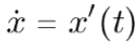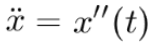# Newton Notation

## Newton Notation for Derivatives

Newton notation for derivatives (which Newton called fluxions) has dots over the function name, instead of primes. The notation is occasionally called “dot notation.”

For example, the first derivative is commonly written in prime notation as a single prime; In Newton notation it would be written with a single dot:Similarly, the second derivative has two dots instead of two primes:## Modern Use

Newton notation for derivatives clearly runs into problems above three or so dots (it runs out of space!). Nor is it convenient when derivatives are taken with respect to more than one variable. Although the notation was fairly widespread historically (especially in England), today it’s mainly used in physics and mechanics where higher order derivatives are rarely needed; one and two dots are sufficient to express velocity and acceleration in basic physics problems (Raleigh, 2007). Newton notation is often used for differentiation with respect to time, whereis more compact and convenient than ds/dt. You may also occasionally see x-dot used for velocities in the occasional physics book (Arianrhod, 2012).

## Newton Notation and the Chain Rule

Although the term “Newton Notation” generally refers to the dotted derivatives outlined above, you may also see the term used to describe other calculus concepts developed by Newton, such as his notation for the chain rule:(Pflueger, 2013).
Which can be written, equivalently (without the small circle—the composition symbol) as:
f(g(x))′ = f′(g(x)) · g′(x) (Magyar, 2020).

## References

Arianrhod, R. (2012). Seduced by Logic: Émilie Du Châtelet, Mary Somerville and the Newtonian Revolution. Oxford University press.
Magyar, P. (2020). The Chain Rule. Retrieved August 24, 2020 from: https://users.math.msu.edu/users/magyarp/Math132-Notes/2.5-Chain-Rule.pdf
Pflueger, N. (2013). Lecture 16: The chain rule. Retrieved August 24, 2020 from: https://npflueger.people.amherst.edu/math1a/lecture16.pdf
Raleigh, S. (2007). Notation Guide for Precalculus and Calculus Students. Retrieved August 24, 2020 from: http://faculty.sdmiramar.edu/sraleigh/Notation%20Guide.pdf

CITE THIS AS:
Stephanie Glen. "Newton Notation" From StatisticsHowTo.com: Elementary Statistics for the rest of us! https://www.statisticshowto.com/newton-notation/